• matplotlibplot函数使用详解

千次阅读 2020-05-26 19:34:59
以上图片中的内容来自于plot函数的帮助文档。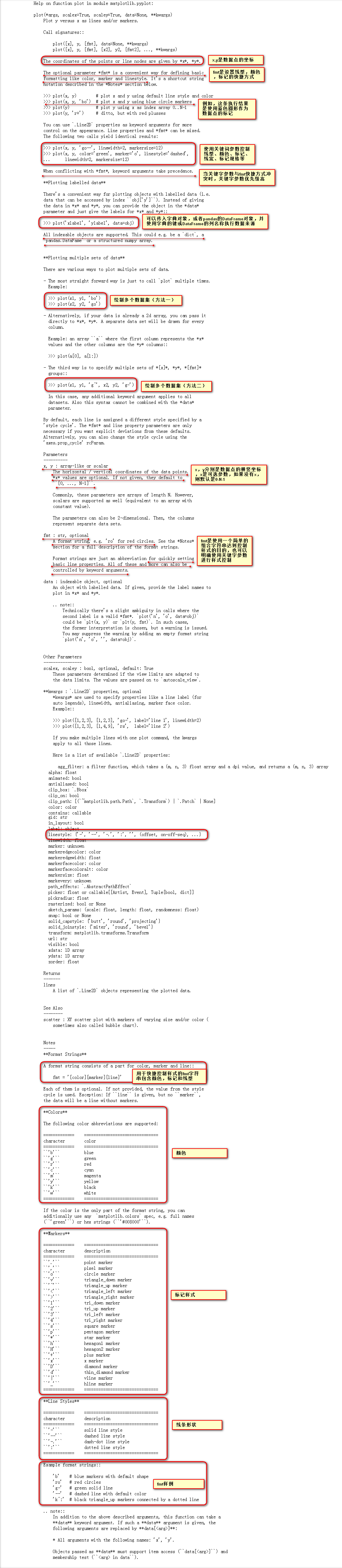以上图片中的内容来自于plot函数的帮助文档。

代码示例：

# 绘制图表，并设置图表的轴刻度，刻度范围，轴标签
# 创建2 x 2的4个子图
fig, axes = plt.subplots(2, 2, figsize=(8, 10), dpi=100)

#数据点
x = np.arange(10)
y = x + np.random.randn(10)

# 第一张子图绘制虚线（红色，数据点使用圆形标记），线宽为2
axes[0, 0].plot(x, y, color="r", linestyle="--", marker="o", linewidth=2)

# 第二张图绘制虚线（蓝绿色，数据点使用+好标记）
axes[0, 1].plot(x, y.cumsum(), "g-.+")

# 第三张绘制散点图（蓝色，数据点使用上三角形标记）
axes[1, 0].scatter(x, y, color="b", marker="^")

# 第四张图绘制散点图（黑色，数据点使用下三角形标记），alpha设置图像的透明度
axes[1, 1].scatter(x, y.cumsum(), color="k", marker="v", alpha=0.3)

# 设置坐标轴的刻度，坐标范围，轴标签
# 默认这些标记在当前的（也就是最后一个）subplot上
plt.xlim([-10, 10])
plt.ylim([-50, 50])
plt.xlabel("axis-x")
plt.ylabel("axis-y")
plt.xticks([-1.0, 0.0, 0.5, 1.5, 2.5, 5, 10])
plt.yticks([-10, 0.0, 5.0, 10.0, 20.0, 30.0, 40.0, 50.0])

# 显式指定每个子图的刻度，坐标范围，轴标签
# 设置第一张子图axes[0, 0]
axes[0, 0].set_xlabel("subplot(0, 0)-x")
axes[0, 0].set_ylabel("subplot(0, 0)-y")
axes[0, 0].set_xlim([-10, 10])
axes[0, 0].set_ylim([-5, 15])
axes[0, 0].set_xticks([-1.0, 0.0, 0.5, 1.5, 2.5, 5, 10])
axes[0, 0].set_yticks([-1.0, 0.0, 0.5, 1.5, 2.5, 5, 10])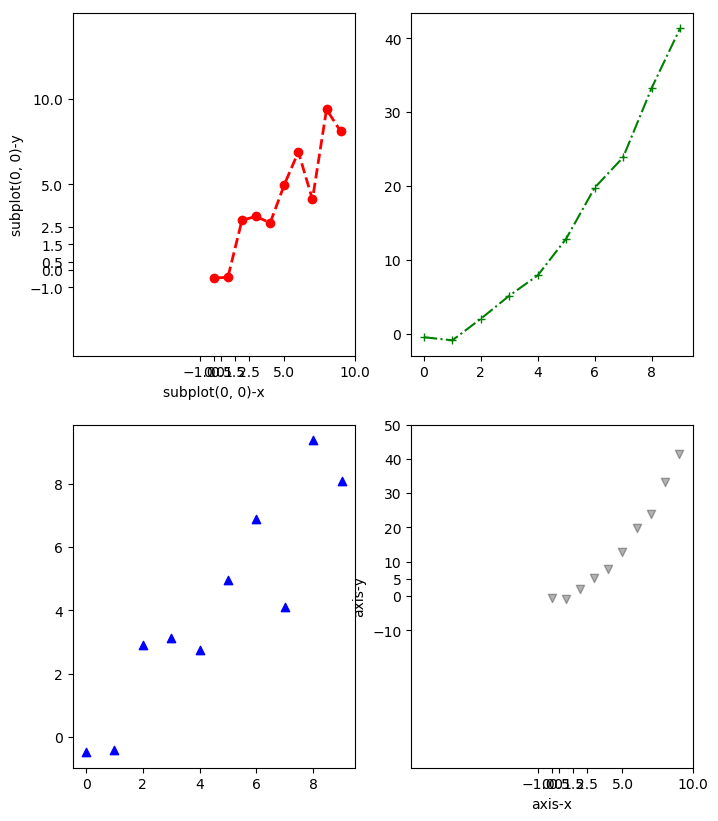展开全文matplotlib plot
• 主要介绍了python matplotlib.pyplot.plot()参数用法，具有很好的参考价值，希望对大家有所帮助。一起跟随小编过来看看吧
• Matplotlib 的用法非常简单，对于最简单的折线图来说，程序只需根据需要给出对应的 X 轴、Y 轴数据，调用 pyplot 子模块下的 plot() 函数即可生成简单的折线图。 假设分析《C语言基础》这本教程从 2013 年到 2019 ...

前言

本文的文字及图片来源于网络,仅供学习、交流使用,不具有任何商业用途,如有问题请及时联系我们以作处理。

PS：如有需要Python学习资料的小伙伴可以加点击下方链接自行获取

python免费学习资料以及群交流解答点击即可加入

Matplotlib 的用法非常简单，对于最简单的折线图来说，程序只需根据需要给出对应的 X 轴、Y 轴数据，调用 pyplot 子模块下的 plot() 函数即可生成简单的折线图。

假设分析《C语言基础》这本教程从 2013 年到 2019 年的销售数据，此时可考虑将年份作为 X 轴数据，将图书各年销量作为 Y 轴数据。程序只要将 2013~2019 年定义成 list 列表作为 X 轴数据，并将对应年份的销量作为 Y 轴数据即可。

例如，使用如下简单的入门程序来展示这套教程从 2013 年到2019 年的销售数据。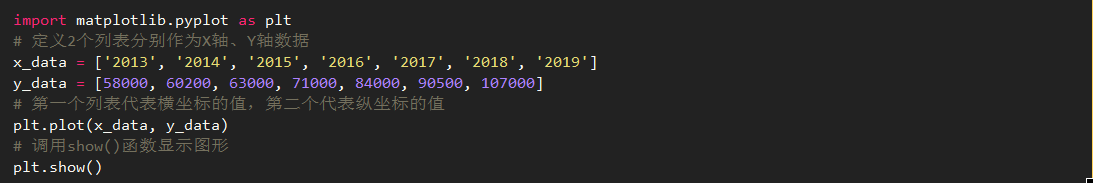上面程序中，第 7 行代码调用 plot() 函数根据 X 轴、Y 轴数据来生成折线图，第 9 行代码则调用 show() 函数将折线图显示出来。

运行上面程序，可以看到生成如图 1 所示的简单折线图。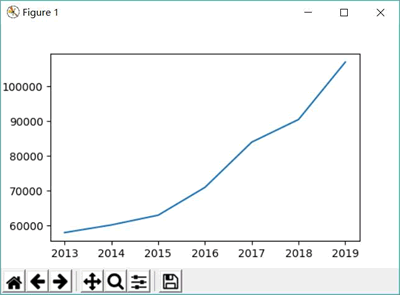如果在调用 plot() 函数时只传入一个 list 列表，该list列表表的数据将作为 Y 轴数据，那么 Matplotlib 会自动使用 0、1、2、3 作为 X 轴数据。例如，将上面程序中的第 7 行代码改为如下形式：

plt.plot(y_data)

再次运行该程序，将看到如图 2 所示的结果。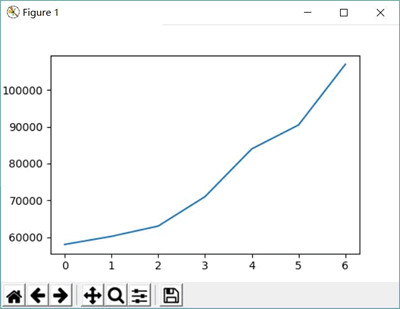plot() 函数除了支持创建具有单条折线的折线图，也支持创建包含多条折线的复式折线图，只要在调用 plot() 函数时传入多个分别代表 X 轴和 Y 轴数据的 list 列表即可。例如如下程序：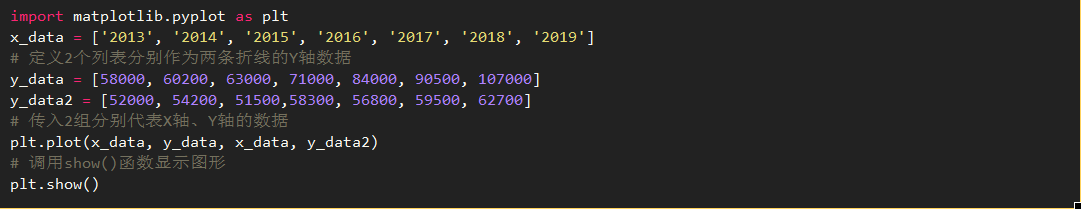上面程序在调用 plot() 函数时，传入了两组分别代表 X 轴、Y 轴数据的 list 列表，因此该程序可以显示两条折线，如图 3 所示。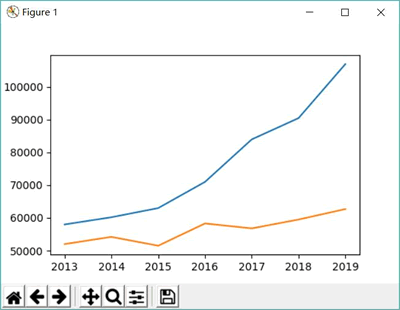图 3 包含多条折线的复式折线图

也可以通过多次调用 plot() 函数来生成多条折线。例如，将上面程序中第 8 行代码改为如下两行代码，程序同样会生成包含两条折线的复式折线图：

plt.plot(x_data, y_data)

plt.plot(x_data, y_data2)

在调用 plot() 函数时还可以传入额外的参数来指定折线的样子，如线宽、颜色、样式等。例如如下程序：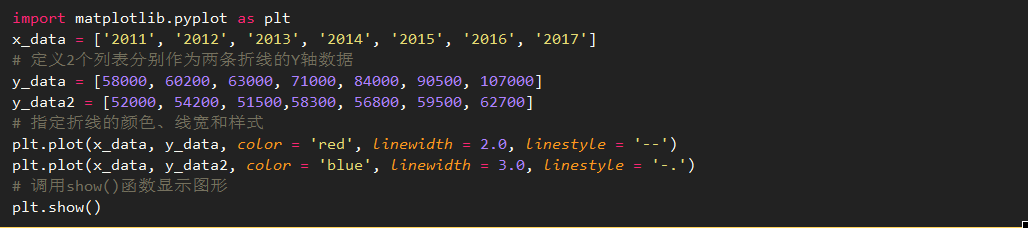上面第 8、9 两行代码分别绘制了两条折线，并通过 color 指定折线的颜色，linewidth 指定线宽，linestyle 指定折线样式。

在使用 linestyle 指定折线样式时，该参数支持如下字符串参数值：

-：代表实线，这是默认值。

--：代表虚线。

·：代表点钱。

-.：代表短线、点相间的虚钱。

运行上面程序，可以看到如图 4 所示的折线图。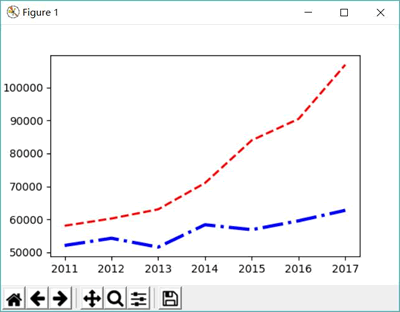展开全文python
• matplotlibplot画图不弹框

千次阅读 2019-08-25 11:16:39
print（plt.plot(np.arange(10))） 原先这么写，就是不弹框，以为是pycharm软件设置问题（差点要去下专业版了），后来试了命令行也不行 最后发现是要调用show（）方法 plt.plot(np.arange(10)) print(plt.show()) ...

print（plt.plot(np.arange(10))）

原先这么写，就是不弹框，以为是pycharm软件设置问题（差点要去下专业版了），后来试了命令行也不行
最后发现是要调用show（）方法

plt.plot(np.arange(10))
print(plt.show())

run一下，成了！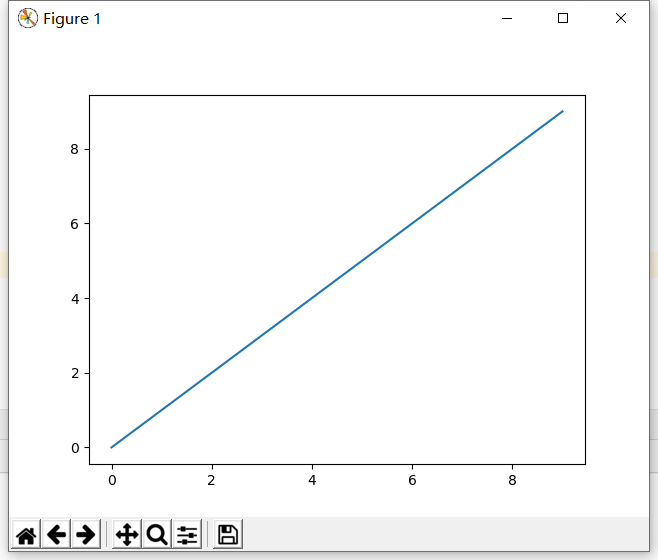展开全文matplotlib python plot
• matplotlibplot保存高清晰图片

千次阅读 2021-02-20 09:31:05
plt.figure(figsize=(10, 5)) plt.savefig('/kaggle/working/feature_importance.png',dpi=600, bbox_inches='tight') plt.show() dpi决定照片的清晰程度
plt.figure(figsize=(10, 5))

plt.savefig('/kaggle/working/feature_importance.png',dpi=600, bbox_inches='tight')
plt.show()

dpi决定照片的清晰程度

展开全文• 主要介绍了matplotlib.pyplot.plot()参数详解，文中通过示例代码介绍的非常详细，对大家的学习或者工作具有一定的参考学习价值，需要的朋友们下面随着小编来一起学习学习吧
• plt.plot()函数是我们平时绘图的时候...matplotlib.pyplot.plot(*args, scalex=True, scaley=True, data=None, **kwargs) #调用格式说明 plot([x], y, [fmt], *, data=None, **kwargs) plot([x], y, [fmt], [x2], y2,数据可视化 python 数据分析
• 在用 matplotlib plot 用 marker 时，如果 marker 超出边界，会被截掉，如： 想让边界上的 marker 也显示完整，在 plot 时设置 clip_on=False，效果： Code import os import numpy as np import seaborn as sns ...matplotlib python
• matplotlib:plot 3d ,plot_surface

千次阅读 2019-01-17 07:44:50
• matplotlib的plot函数说明

千次阅读 2017-08-18 15:24:23
matplotlib.pyplot.plot(*args, **kwargs): 绘制线和/或标记到Axex(轴)。 args是一个可变长度参数，允许使用可选格式字符串的多个x，y对。  例如，以下每个都是合法的： plot(x, y) # 使用默认line风格与颜色绘制x...plot函数
• Matplotlib plot 绘制简单折线图 1. example 1 import matplotlib.pyplot as plt squares = [1, 4, 9, 16, 25] plt.plot(squares) plt.show() 2. example 2 # example 2 import matplotlib.pyplot as plt...plot
• matplotlib.pyplot.plot()参数详解

千次阅读 2019-05-08 21:01:13
matplotlib.pyplot.plot(*args,**kwargs) 绘制线条或标记的轴。参数是一个可变长度参数，允许多个X、Y对可选的格式字符串。 例如，下面的每一个都是合法的： plot(x, y) #plot x, y使用默认的线条...
• plt.plot(x, y, 'xxx', label=, linewidth=) 参数1：位置参数，点的横坐标，可迭代对象 参数2：位置参数，点的纵坐标，可迭代对象 参数3：位置参数，点和线的样式，字符串 此参数分三部分，点线的颜色、点的形状...Python plot
• plt.plot(x,y,ls="-",label=“x”)，也有写成plot（x,y,**kwargs） x:x轴数值 y:y轴数值 ls:折线图的线条风格 也可以用kind="–g^",其中g表示的颜色的缩写,符号表示的是虚线和和符号的组合 kind属性包含了 linestyle...
• %matplotlib qt5 import matplotlib.pyplot as plt import numpy as np # 解决汉字乱码 import matplotlib as mpl # 字体会按照列表依次从电脑中查找,直到找到 plt.rcParams['font.family'] = ['Arial Unicode MS','...
• Matplotlib之plot.show方法

万次阅读 2018-07-16 16:52:46
在使用Matplotlib画图时： 尽量不要使用show方法，不到万不得已千万别用，阻塞函数。 可以使用pause函数代替show。pause可以再一段时间间隔内刷新，并显示GUI界面，主要是不阻塞。...python matplotlib
• 介绍了matplotlib的基本应用方法，部分有美化介绍，可以作为使用时的一个参考
• 使用plt.imshow()显示的时候要注意，设置cmap参数为‘gray’ 第一种方法，使用PIL的Image模块 from PIL import Image lena_gray = Image.open('lena.jpg').convert('L') plt.imshow(lena_gray, cmap='gray') ...
• 今天小编就为大家分享一篇Python matplotlib通过plt.scatter画空心圆标记出特定的点方法，具有很好的参考价值，希望对大家有所帮助。一起跟随小编过来看看吧
• matplotlib plot 绘图函数发生阻塞的解决办法。plot 阻塞
• 欢迎关注”生信修炼手册”!在matplotlib中，plot命令主要用于绘制折线图，基本用法如下>>> importmatplotlib.pyplot asplt...数据可视化 css 数据分析 编程语言 python
• matplotlibplot绘图简介

千次阅读 2017-09-16 22:34:34
• 解决方法主要来自stackoverflow： python-plotting-on-remote-server-...remote-debugging-with-pycharm-unable-plot-figure-on-local-machine Ok, after some more googling I finally managed to get this...
• Matplotlib的画图功能是数据分析里面非常好用的一款工具，这款工具可以做到将庞杂的实验数据用以图表的形式简单直观的显示出来,由于之后的学习过程中会频频使用到数据进行实验,掌握了这款工具之后的学习可以便利很多...matplotlib python ubuntu
• Matplotlib学习笔记（一）：plot()函数Python Matplotlib plot
• Matplotlib - 折线图 plot() 所有用法详解

万次阅读 多人点赞 2019-02-15 18:09:03
散点图和折线图是数据分析中最常用的两种图形。...Matplotlib 中绘制散点图的函数为 plot() ，使用语法如下：matplotlib.pyplot.plot(*args, scalex=True, scaley=True, data=None, **kwargs)...Matplotlib plot 折线图
• Python 用 matplotlib 中的 plot 画图，折线图

万次阅读 多人点赞 2019-08-22 21:14:47
文章目录1. 加载宏包2. plot 函数的语法plot([x], y, [fmt], **kwargs)3. 一张图画好几条线 1. 加载宏包 使用 matplotlib 包...# 导入 matplotlib 中的 plot， 并命名为常用名 plt import matplotlib.pyplot as p...python plot matplotlib pyplot
• 在用目标检测API进行模型测试时，测试... 查阅资料发现出现这种情况一般有两种原因，一是调用图片显示的语法不对，第二种是跟matplotlib的后端有关,还有一种是调用的库的模块不同导致的。 1.1 调用图片显示的语法不...
• import matplotlib.pyplot as plt 基本语法： plt.plot(x, y, format_string, **kwargs) x：x轴数据，列表或数组，可选 y：y轴数据，列表或数组 format_string：控制曲线的格式字符串，可选，如不写为默认格式 color......